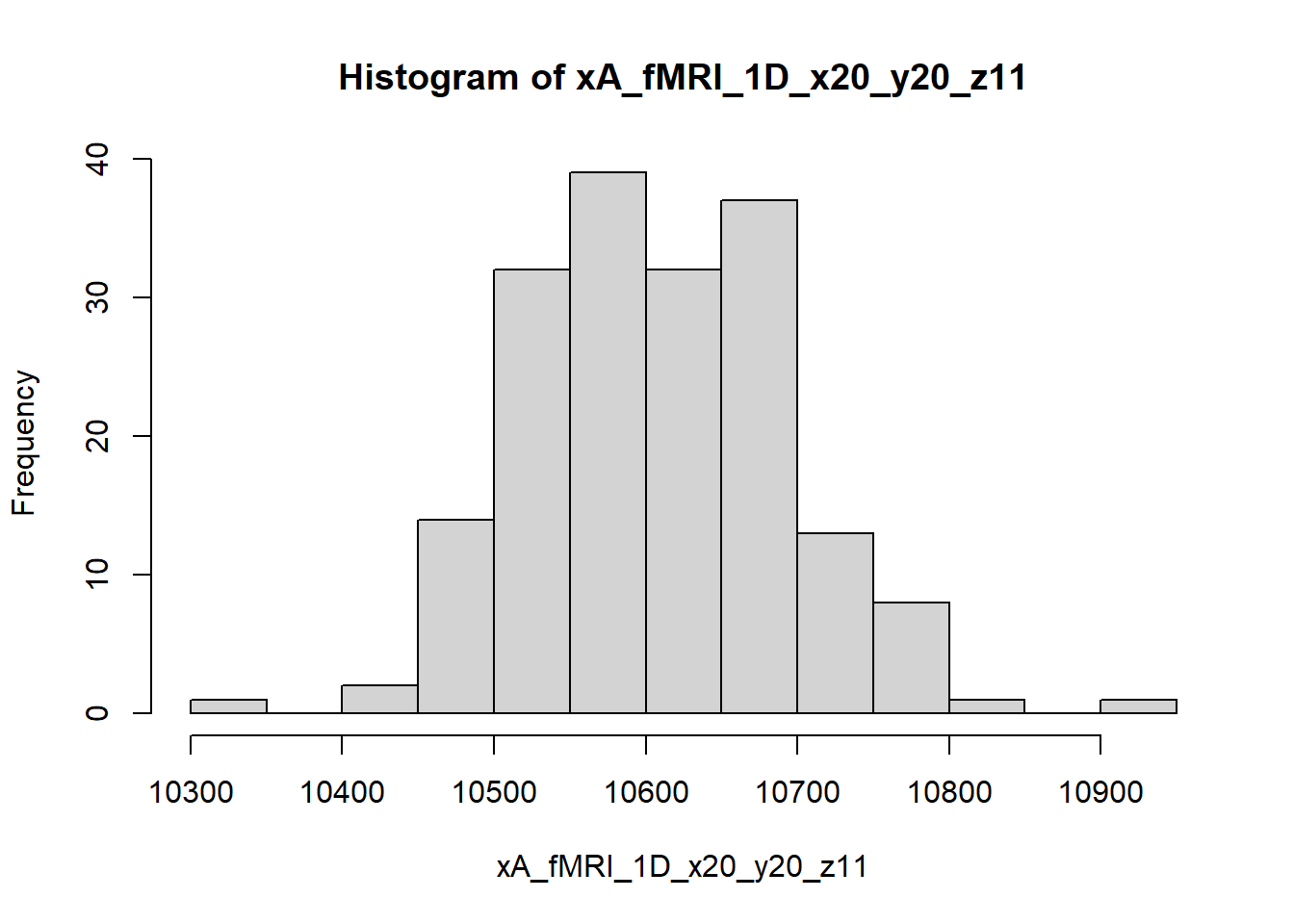# 1 Spacetime IID vs. Spacekime Sampling

For all natural spacetime processes, various population characteristics like the mean, variance, range, and quantiles can be estimated by collecting independent and identically distributed (IID) samples. These samples represent observed data that is traditionally used to obtain sample-driven estimates of the specific population characteristics via standard formulas like the sample arithmetic average, variance, range, quantiles, etc. The latter approximate their population counterparts and form the basis for classical parametric and non-parametric statistical inference.

Typically, reliable spacetime statistical inference is conditional on the distribution of the native process as well as a sample-size reflecting the characteristics of the phenomenon. We will demonstrate that spacekime analytics can be equally effective with measuring a single spacetime observation and having a reasonable estimates of the unobserved process kime-phases.

Without loss of generality, suppose we have a pair of cohorts \(A\) and \(B\) and we obtain a series of measurements \(\{X_{A,i}\}_{i=1}^{n_A}\) and \(\{X_{B,i}\}_{i=1}^{n_B}\), respectively. Obviously the relations between the cohorts could widely vary, from being samples of the same process, to being related or completely independent.

To allow us to examine the extreme cases of pairing (1) IID cohorts (\(A\) and \(B\)), and (2) independent but differently distributed cohorts (\(A\) and \(C\)). The latter case may be thought of as a split of the first cohort (\(A\)) into training (\(C\)) and testing (\(D\)) groups. This design allows us to compare the classical spacetime-derived population characteristics of cohort \(A\) to their spacekime-reconstructed counterparts obtained using a single random kime-magnitude observation from \(A\) and kime-phases estimates derived from cohorts \(B\), \(C\) or \(D\).

## 1.1 fMRI timeseries

The demonstration below is based on a functional magnetic resonance imaging (fMRI) data, which is a 4D hypervolume with intensities representing the blood oxygenation level dependence at a specific spacetime location \((x,y,z,t)\). For simplicity, we will only focus on two fixed spatial locations with varying intensity distributions.

``````library(EBImage)
require(brainR)
library(spatstat)
library(ggplot2)
library(kSamples)
library(reshape2)
library(beanplot)
library(rstanarm)

fMRIURL <- "http://socr.umich.edu/HTML5/BrainViewer/data/fMRI_FilteredData_4D.nii.gz"
fMRIFile <- file.path(tempdir(), "fMRI_FilteredData_4D.nii.gz")
# dimensions: 64 x 64 x 21 x 180 ; 4mm x 4mm x 6mm x 3 sec

fMRIVolDims <- dim(fMRIVolume); # fMRIVolDims
# time_dim <- fMRIVolDims; time_dim ## 180

# 2. extract the time-corse of 1D mid-axial slice (3D) hypervolume
xA_fMRI_1D_x20_y20_z11 <- fMRIVolume[20, 20, 11, ]; # length(xA_fMRI_1D_x20_y20_z11)   #  180
hist(xA_fMRI_1D_x20_y20_z11)````````````xB_fMRI_1D_x30_y30_z13 <- fMRIVolume[30, 30, 13, ]; # length(xB_fMRI_1D_x30_y30_z13)   #  180
# hist(xB_fMRI_1D_x30_y30_z13)

# Now, combine your two 1D timeseries into one dataframe for joint hist plotting as densities.
# First make a new column in each that will be
# a variable to identify where they came from later.
xA_df <- as.data.frame(xA_fMRI_1D_x20_y20_z11)
colnames(xA_df) <- "value"; xA_df\$cohort <- "xA"
xB_df <- as.data.frame(xB_fMRI_1D_x30_y30_z13)
colnames(xB_df) <- "value"; xB_df\$cohort <- "xB"

# and combine into your new data frame vegLengths
xA_xB_df <- rbind(xA_df, xB_df)

ggplot(xA_xB_df, aes(value, fill = cohort)) +
geom_density(alpha = 0.5, size=1.2) +
theme(text = element_text(size=20)) +
xlim(c(10200, 12000))``````